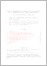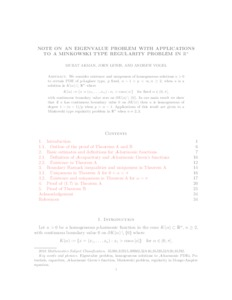# Note on an eigenvalue problem with applications to a Minkowski type regularity problem in Rⁿ

Akman, Murat and Lewis, John and Vogel, Andrew (2019) 'Note on an eigenvalue problem with applications to a Minkowski type regularity problem in Rⁿ.' Calculus of Variations and Partial Differential Equations, 59 (2). ISSN 0944-2669 (In Press)Preview
Text
ALV Final.pdf - Submitted Version

We consider existence and uniqueness of homogeneous solutions u > 0 to certain PDE of p-Laplace type, p fixed, n - 1 < p <∞, n ≥ 2, when u is a solution in K(α) ⊂ Rⁿ where K(α) := { x = (x₁,..., x_n ) : x₁ > cos α | x| } for fixed α ∈ (0, π ], with continuous boundary value zero on ∂K (α) \ {0\}. In our main result we show that if u has continuous boundary value 0 on ∂K (π)$then u is homogeneous of degree 1 - (n-1)/p when p > n - 1. Applications of this result are given to a Minkowski type regularity problem in Rⁿ when n = 2; 3. Item Type: Article Eigenvalue problem; homogeneous solutions to$\mathcal{A}$-harmonic PDEs; Potentials, capacities,$\mathcal{A}\$-harmonic Green's function, Minkowski problem, regularity in Monge-Amp{\`e}re equation Faculty of Science and HealthFaculty of Science and Health > Mathematical Sciences, Department of Elements Elements 12 Sep 2019 13:05 26 Feb 2022 10:40 http://repository.essex.ac.uk/id/eprint/25322View Item